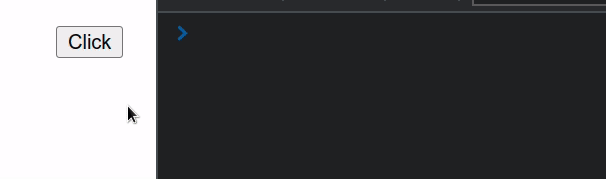# 迹忆客专注技术分享

## 在 React 中调用多个 onClick 函数

1. 在元素上设置 onClick 属性。
2. 在事件处理函数中调用其他函数。
3. 事件处理函数可以根据需要调用尽可能多的其他函数。
``````export default function App() {
const sum = (a, b) => {
return a + b;
};

const multiply = (a, b) => {
return a * b;
};

return (
<div>
<button
onClick={event => {
console.log('function 1:', sum(5, 5));
console.log('function 2:', multiply(5, 5));
}}
>
Click
</button>
</div>
);
}
````````````<button
onClick={event => {
console.log('function 1:', sum(5, 5));
console.log('function 2:', multiply(5, 5));
}}
>
Click
</button>
``````

## 在 JSX 代码之外提取事件处理程序

``````export default function App() {
const sum = (a, b) => {
return a + b;
};

const multiply = (a, b) => {
return a * b;
};

const handleClick = event => {
console.log(event.target);

console.log('function 1:', sum(5, 5));
console.log('function 2:', multiply(5, 5));
};

return (
<div>
<button onClick={handleClick}>Click</button>
</div>
);
}
````````````const handleClick = event => {
console.log(event.target);

console.log('function 1:', sum(5, 5));
console.log('function 2:', multiply(5, 5));
};
``````

`请注意` ，我们正在将一个函数传递给 onClick 属性，而不是调用一个函数的结果。

``````<button onClick={handleClick}>Click</button>
``````

## 相关文章

### React 中在 map() 中使用条件跳出map

React 中在 map() 中使用条件： 在数组上调用 map() 方法。 使用 if 条件，如果条件满足则显式返回。 否则返回不同的值或返回 null 以不呈现任何内容。 export default function App () { const arr =

### 在 React 中按类名查找所有元素## 热门标签